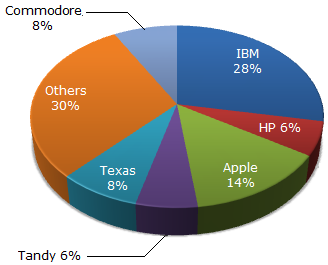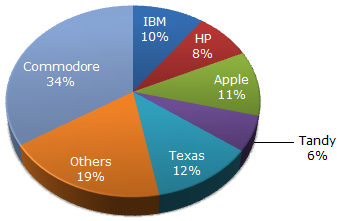# Data Interpretation - Pie Charts

### Exercise :: Pie Charts - Pie Chart 4

The pie chart shows the distribution of New York market share by value of different computer companies in 2005.The pie chart shows the distribution of New York market share by volume of different computer companies in 2005.

Number of units sold in 2005 in New York = 1,500

Value of units sold in 2005 in New York = US \$1,650,000.1.

For the year 2005, which company has realised the lowest average unit sales price for a PC ?

 A. Commodore B. IBM C. Tandy D. Cannot be determined

Explanation:

Although it seems to be Commodore, the answer cannot be determined due to the fact that we are unaware of the break-up of the sales value and volume of companies compromising the other categories.

2.

Over the period 2005-2006, if sales (value-wise) of IBM PC's increased by 50% and of Apple by 15% assuming that PC sales of all other computer companies remained the same, by what percentage (approximately) would the PC sales in New York (value-wise) increase over the same period ?

 A. 16.1 % B. 18 % C. 14 % D. None of these

Explanation:

If we assume the total sales to be 100 in the first year, IBM's sales would go up by 50% (from 28 to 42) contributing an increase of 14 to the total sales value.

Similarly, Apple's increase of 15% would contribute an increase of 2.1 to the total sales value. The net change would be 14 + 2.1 on 100. (i.e., 16.1%)

3.

In 2005, the average unit sale price of an IBM PC was approximately (in US\$)

 A. 3180 B. 2800 C. 393 D. 3080

Explanation:

IBM accounts for 28% of the share by value and 10% of the share by volume.

28% of 1650000 = 28 x 1650000/100 = 462000

10% of 1500 = 10 x 1500/100 = 150

Therefore, average unit sale price = 462000/150 = 3080.